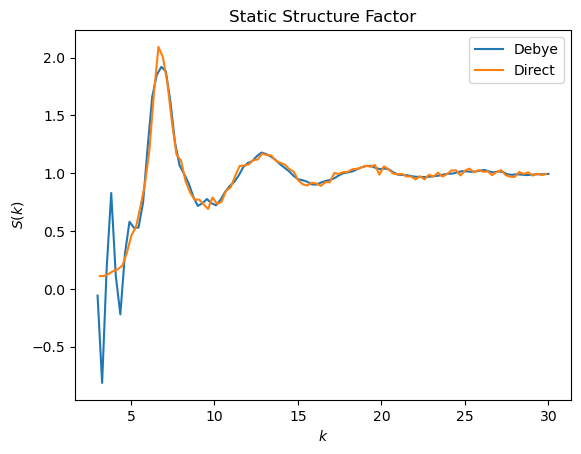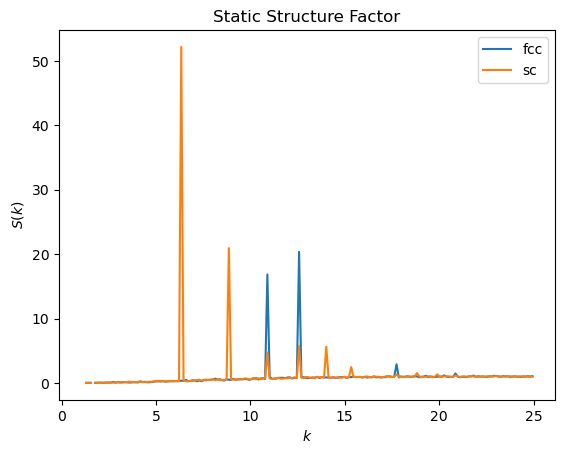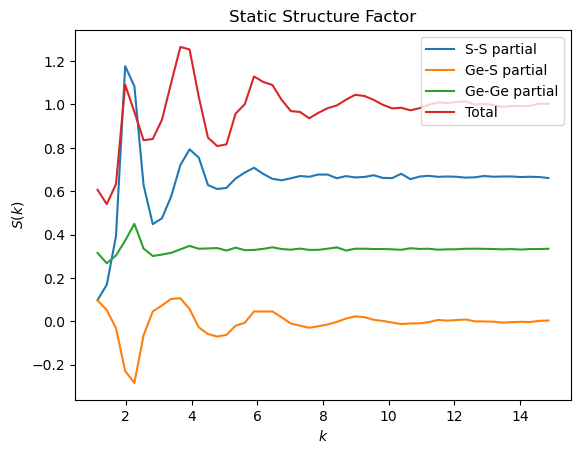# freud.diffraction.StaticStructureFactorDirect and freud.diffraction.StaticStructureFactorDebye#

The freud.diffraction module provides two methods for calculating a one-dimensional static structure factor $$S(k)$$ which can be used to characterize structure of crystals, liquids or amorphous phases.

The freud.diffraction.StaticStructureFactorDirect class implements a “direct” $$S(k)$$ method. First, the following expression is computed over a $$k$$-space (reciprocal space) grid:

$S(\vec{k}) = {\frac{1}{N}}\sum_{i=1}^{N}\sum_{j=1}^{N}\mathrm{e}^{-i\vec{k}\cdot(\vec{r}_{i} - \vec{r}_{j})}$

Then, the angular dependence is integrated out, resulting in $$S(|\vec{k}|)$$, otherwise denoted $$S(k)$$. For an excellent introduction to the theory of scattering and $$S(k)$$, please refer to the documentation of the dynasor package, which performs a number of calculations related to scattering. The freud library implements the core method of static structure factor calculation based on the dynasor package, with some additional performance optimizations in parallelized C++ code, as well as an interface to compute $$S(k)$$ that aligns with the APIs and conventions of the freud library.

The freud.diffraction.StaticStructureFactorDebye class computes static structure factor based on the Debye scattering equation:

$S(k) = {\frac{1}{N}} \sum_{i=1}^{N}\sum_{j=1}^{N}{\frac{\sin(kr_{ij})}{kr_{ij}}}$

which is obtained by integrating out the angular dependence from the original formula. This implementation provides a much faster algorithm, but gives worse results than the “direct” method at low $$k$$ values.

Note that freud employs the usual physics convention, as opposed to the crystallographic convention, with the following expression linking the two: $$k = 2\pi q$$. The static structure factor is related to the radial distribution function, $$g(r)$$, by a Fourier Transform:

$S(k) = 1 + \rho \int_{V}\mathrm{d}\vec{r}e^{-i\vec{k}\cdot\vec{r}}g(r).$

## Lennard-Jones Liquid Example#

One of the use cases for $$S(k)$$ is to characterize structure of liquids. The example shown here uses data generated by a HOOMD-blue simulation of a 1000 particle system subject to the Lennard-Jones potential. See the HOOMD-blue documentation and examples for more information.

:

import freud
import gsd.hoomd
import matplotlib.pyplot as plt
import numpy as np

bins = 100
k_max = 30
k_min = 3
sfDirect = freud.diffraction.StaticStructureFactorDirect(
bins=bins, k_max=k_max, k_min=k_min
)
sfDebye = freud.diffraction.StaticStructureFactorDebye(
num_k_values=bins, k_max=k_max, k_min=k_min
)

with gsd.hoomd.open("data/LJsampletraj.gsd", "rb") as traj:
for frame in traj:
sfDebye.compute(frame, reset=False)
sfDirect.compute(frame, reset=False)

plt.plot(sfDebye.k_values, sfDebye.S_k, label="Debye")
plt.plot(sfDirect.bin_centers, sfDirect.S_k, label="Direct")
plt.title("Static Structure Factor")
plt.xlabel("$k$")
plt.ylabel("$S(k)$")
plt.legend()
plt.show()## Crystal Comparison Example#

The static structure factor $$S(k)$$ can also be used to characterize and compare crystal structures. In the below example we compare the computed static structure factors $$S(k)$$ of a face-centered cubic (fcc) crystal and simple cubic (sc) crystal.

:

sf = freud.diffraction.StaticStructureFactorDirect(bins=200, k_max=25, k_min=1)
fcc_system = freud.data.UnitCell.fcc().generate_system(10, sigma_noise=0.10)
sf.compute(fcc_system)
plt.plot(sf.bin_centers, sf.S_k, label="fcc")

sc_system = freud.data.UnitCell.sc().generate_system(10, sigma_noise=0.10)
sf.compute(sc_system)
plt.plot(sf.bin_centers, sf.S_k, label="sc")

plt.title("Static Structure Factor")
plt.xlabel("$k$")
plt.ylabel("$S(k)$")
plt.legend()
plt.show()## Calculation of Partial Structure Factors#

Both methods support calculation of partial structure factors according to Faber-Ziman decomposition. In the conventions adopted in freud, the summation of partials reproduces the total scattering. In this example we load a simulation trajectory of $$\text{GeS}_2$$ and calculate the Ge-Ge partial, the S-S partial and the mixed Ge-S partial (which is the same as S-Ge partial). The calculation of the partials requires the usage of query_points and N_total parameters for the compute method.

:

import freud
import matplotlib.pyplot as plt
import numpy as np

N_particles = int(np.genfromtxt("data/ges2.xyz", max_rows=1, dtype=int))
cleaned_data = []
# remove lines that don't contain particle data
with open("data/ges2.xyz") as f:
for line in f:
if line != "\n" and line != " ":
cleaned_data.append(line)

positions = np.genfromtxt(cleaned_data)[:, 1:4].reshape(-1, N_particles, 3)
particle_types = np.genfromtxt(cleaned_data, dtype=str)[:, 0].reshape(-1, N_particles)

box = freud.Box.cube(19.21)

# max_k_points is the number of k-points used in the calculation,
# higher values give better S(k) but takes longer
k_max = 15
k_min = 1
bins = 50
sfGe_Ge = freud.diffraction.StaticStructureFactorDirect(
bins=bins, k_max=k_max, k_min=k_min
)
sfGe_S = freud.diffraction.StaticStructureFactorDirect(
bins=bins, k_max=k_max, k_min=k_min
)
sfS_S = freud.diffraction.StaticStructureFactorDirect(
bins=bins, k_max=k_max, k_min=k_min
)
sfTotal = freud.diffraction.StaticStructureFactorDirect(
bins=bins, k_max=k_max, k_min=k_min
)

for frame_positions, frame_types in zip(positions, particle_types):
Ge_positions = frame_positions[frame_types == "Ge"]
S_positions = frame_positions[frame_types == "S"]
sfGe_Ge.compute(
(box, Ge_positions),
query_points=Ge_positions,
N_total=N_particles,
reset=False,
)
sfGe_S.compute(
(box, S_positions),
query_points=Ge_positions,
N_total=N_particles,
reset=False,
)
sfS_S.compute(
(box, S_positions),
query_points=S_positions,
N_total=N_particles,
reset=False,
)
sfTotal.compute(
(box, frame_positions),
reset=False,
)

plt.plot(sfS_S.bin_centers, sfS_S.S_k, label="S-S partial")
plt.plot(sfGe_S.bin_centers, sfGe_S.S_k, label="Ge-S partial")
plt.plot(sfGe_Ge.bin_centers, sfGe_Ge.S_k, label="Ge-Ge partial")

# Note that the Ge-S partial must be included twice
S_tot = sfS_S.S_k + 2 * sfGe_S.S_k + sfGe_Ge.S_k
assert np.allclose(S_tot, sfTotal.S_k, atol=1e-5, rtol=1e-5)

plt.plot(sfGe_Ge.bin_centers, S_tot, label="Total")
plt.title("Static Structure Factor")
plt.xlabel("$k$")
plt.ylabel("$S(k)$")
plt.legend(loc="upper right")
plt.show()